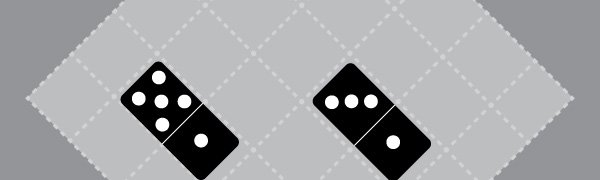# Blocking Dominos

Probability Level 4Diego wants to completely cover a $6 \times 6$ board using 18 dominos. Find the smallest positive integer $k$ which satisfies the following property:

It is possible for Diego to place $k$ dominos, such that there is a unique way to completely cover the remaining squares.

×